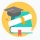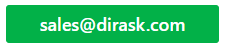Home
IT Knowledge
IT Career
Inspiration
Languages
EN

# JavaScript - binary search algorithm example

11 points
Created by:AnnLen
9180

## 1. Overview

Binary search is fast search algorithm with run-time complexity `O(log n)`. This algorithm requires to work on sorted data with random access to each element.

Main idea of binary search algorithm is to:

1. find middle point index in actual range with formula:
`var point = Math.ceil((index + limit) / 2);`
Notes:
• middle point index has been marked with black array on below image,
• at begining actual range contains all array elements (`index=0`, `limit=array.length-1`),
2. if middle point value is equal to expected value algorithm returns index,
3. if middle point value is not equal to expected value, range is splitted into left or right ranges with excluded middle point,

Algorithm iterations:

## 2. Custom iterative implementation example

``````// ONLINE-RUNNER:browser;

function searchIndex(array, value) {
var index = 0;
var limit = array.length - 1;

while (index <= limit) {
var point = Math.ceil((index + limit) / 2);
var entry = array[point];

if (value > entry) {
index = point + 1;
continue;
}

if (value < entry) {
limit = point - 1;
continue;
}

return point; // value == entry
}

return -1;
}

// Example:

// for this implementation array must be sorted from smallest to biggest!
var array = [ 4, 5, 7, 11, 12, 15, 15, 21, 40, 45 ];
var index = searchIndex(array, 11);

console.log(index); // 3``````

## 3. Custom recursive implementation example

``````// ONLINE-RUNNER:browser;

function searchIndex(array, value) {
function split(index, limit) {
if (index > limit) {
return -1;
}

var point = Math.ceil((limit + index) / 2);
var entry = array[point];

if (value < entry) {
return split(index, point - 1);
}

if (value > entry) {
return split(point + 1, limit);
}

return point; // value == entry
}

return split(0, array.length - 1);
}

// Example:

// for this implementation array must be sorted from smallest to biggest!
var array = [ 4, 5, 7, 11, 12, 15, 15, 21, 40, 45 ];
var index = searchIndex(array, 11);

console.log(index); // 3``````